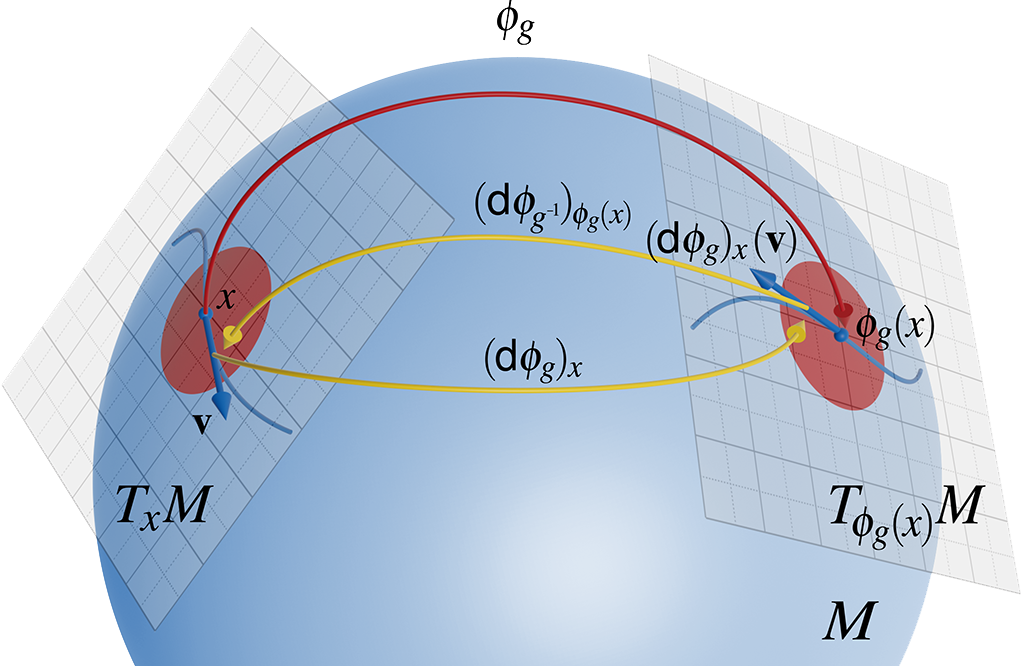## Tutorial on Riemannian Geometry for Scientific Visualization (2022)

#### Markus Hadwiger, Thomas Theußl, Peter Rautek### Abstract

This tutorial introduces the most important basics of Riemannian geometry and related concepts with a specific focus on applications in scientific visualization. The main concept in Riemannian geometry is the presence of a Riemannian metric on a differentiable manifold, comprising a second-order tensor field that defines an inner product in each tangent space that varies smoothly from point to point. Technically, the metric is what allows defining and computing distances and angles in a coordinate-independent manner. However, even more importantly, it in a sense is really the major structure (on top of topological considerations) that defines the space where scientific data, such as scalar, vector, and tensor fields live.

However, the concept of a metric, and crucial related concepts such as connections and covariant derivatives, are not often used explicitly in visualization. In contrast to concepts of differential topology, which have been used extensively in visualization, for example in scalar and vector field topology, we believe that concepts from Riemannian geometry have been underrepresented in the visualization literature. One reason for this might be that most visualization techniques are developed for scalar, vector, or tensor fields given in Euclidean space $$\mathbb{R}^2$$ or $$\mathbb{R}^3$$, and data given on curved surfaces are usually treated explicitly through their embedding in $$\mathbb{R}^3$$. However, the presence of a Riemannian metric on a manifold has very important implications even for data given in Euclidean space, for example regarding the physical meaning of visualizations as well as for the use of non-Cartesian coordinates. Therefore, considering the metric tensor field explicitly provides several important benefits.

### Objectives

In this tutorial, we try to particularly highlight the additional insight that can be gained from employing concepts from Riemannian geometry in scientific visualization. However, although we believe that insight is the most important benefit to be gained from using these concepts, we also discuss computational advantages. In addition to Riemannian metrics, we also introduce the most important related concepts from modern, coordinate-free differential geometry, in particular general (non-Cartesian) tensor fields and differential forms, smooth mappings between manifolds, Lie derivatives, and Lie groups and Lie algebras. Throughout the tutorial, we use several examples from the scientific visualization literature, dealing with scalar, vector, or tensor fields, respectively, and highlight their implicit or explicit connections to Riemannian geometry.

### Motivation and Audience

While there exist a lot of general mathematical textbooks and courses on differential geometry and Riemannian geometry, we are not aware of any course that specifically targets visualization researchers and practitioners. Furthermore, the concepts—and in particular the emphasis—most relevant and important for visualization techniques are hard to extract from standard geometry texts, which often cover a large amount of advanced material. At the same time, time-dependent data, such as unsteady vector fields, are not treated in sufficient detail in most geometry texts. This tutorial aims to start filling this gap for researchers and practitioners in visualization, on an intermediate level.

### Speakers